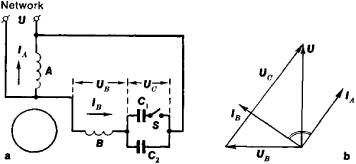# Asynchronous Capacitor Motor

The following article is from The Great Soviet Encyclopedia (1979). It might be outdated or ideologically biased.

## Asynchronous Capacitor Motor

(1) An asynchronous electric motor with single-phase mains, having two windings on its stator; one winding is connected directly to the power supply, and the other is connected in series with a capacitor to generate a rotating magnetic field. The capacitors cause a phase shift between the currents flowing through windings A and B (Figure 1), whose axes are spatially displaced. Maximum torque is developed when the phase shift between the two currents is 90° and the amplitude of the currents is selected such that the rotating field becomes circular.

To start an asychronous capacitor motor both capacitors (C1 and C2) are connected to the circuit; after the run-up, one capacitor (for example, C1) is disconnected, since operation at rated speed requires considerably less capacitance than the startup. The start-up and operating characteristics of an asynchronous capacitor motor are close to those of a three-phase asychronous motor. Asynchronous capacitor motors are used in low-power electric drives; if the power rating exceeds 1 kilowattFigure 1. Circuit (a) and vector diagram (b) of an asynchronous capacitor motor:(U), (UB), and (UC) voltages; (IA) and (IB) currents; (A) and (B) stator windings; (S) centrifugal switch that disconnects capacitor C1, after run-up of the motor; (C1) and (C2) capacitorsFigure 2. Diagram of connection of a three-phase asynchronous motor to a single-phase network: (a) star-connected stator windings, (b) delta-connected stator windings; (S1) and (S2) switches, (Cop) operating capacitor, (Cs) starting capacitor, (M) asynchronous electric motor

they are seldom used, because of the cost and size of the capacitors.

(2) A three-phase asynchronous electric motor, connected through a capacitor to single-phase mains. The operating capacitance of the capacitor for a three-phase motor is determined from the formula Cop= 2,800 (I/U) (microfarads) if the windings are star-connected and from the formula Cop = 4,800 (I/U) (microfarads) if the windings are delta-connected (Figure 2). The capacitance of the starting capacitor Cs is (2.5–3.0)Cop. The operating voltage of the capacitors must be 1.5 times that of the mains voltage; paper capacitors must be used.

The Great Soviet Encyclopedia, 3rd Edition (1970-1979). © 2010 The Gale Group, Inc. All rights reserved.
Site: Follow: Share:
Open / Close